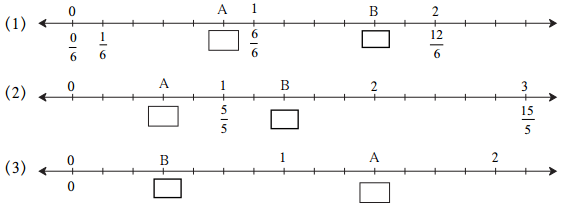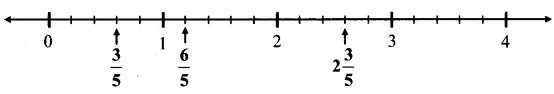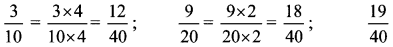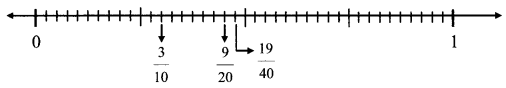# Maharashtra Board Practice Set 11 Class 6 Maths Solutions Chapter 4 Operations on Fractions

Balbharti Maharashtra State Board Class 6 Maths Solutions covers the Std 6 Maths Chapter 4 Operations on Fractions Class 6 Practice Set 11 Answers Solutions.

## 6th Standard Maths Practice Set 11 Answers Chapter 4 Operations on Fractions

Question 11.
What fractions do the points A and B show on the number lines below?Solution:
(1) Each unit is divided in 6 parts
A is 5th division from 0
∴ $$A=\frac { 5 }{ 6 }$$

B is 10th division from 0
∴ $$B=\frac { 10 }{ 6 }$$

(2) Each unit is divided in 5 parts
A is 3rd division from 0
∴ $$A=\frac { 3 }{ 5 }$$

B is 7th division from 0
∴ $$B=\frac { 7 }{ 5 }$$

(3) Each unit is divided in 7 parts
A is 10th division from 0
∴ $$A=\frac { 10 }{ 7 }$$

B is 3rd division from 0
∴ $$B=\frac { 3 }{ 7 }$$

Question 2.
Show the following fractions on the number line:
i. $$\frac{3}{5}, \frac{6}{5}, 2 \frac{3}{5}$$
ii. $$\frac{3}{4}, \frac{5}{4}, 2 \frac{1}{4}$$
Solution:
i. $$\frac{3}{5}, \frac{6}{5}, 2 \frac{3}{5}$$ii. $$\frac{3}{4}, \frac{5}{4}, 2 \frac{1}{4}$$Maharashtra Board Class 6 Maths Chapter 4 Operations on Fractions Practice Set 11 Intext Questions and Activities

Question 1.
If we want to show the fractions $$\frac{3}{10}, \frac{9}{20}, \frac{19}{40}$$ on the number line, how big should the unit be? (Textbook pg. no. 24)
Solution:
The denominators of the given fractions are not equal.
The numbers in the denominators 10, 20 and 40 have common multiple 40.
∴ Making the denominators equal, we get∴ To represent these fractions on the numbers line, each main unit should be divided into 40 equal sub-units.Therefore,
$$\frac{3}{10}=\frac{12}{40}$$ is represented on 12th mark from 0.
$$\frac{9}{20}=\frac{18}{40}$$ is represented on 18th mark from 0 and
$$\frac { 19 }{ 40 }$$ is represented on 19 mark from 0.

Scroll to Top